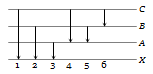The figure indicates the energy level diagram of an atom and the origin of six spectral lines in emission (e.g. line no. 5 arises from the transition from level B to A). The following spectral lines will also occur in the absorption spectrum(a) 1, 4, 6                      (b) 4, 5, 6
(c) 1, 2, 3                      (d) 1, 2, 3, 4, 5, 6

Concept Questions :-

Spectral series
High Yielding Test Series + Question Bank - NEET 2020

Difficulty Level:

When a hydrogen atom is raised from the ground state to an excited state

(a) P.E. increases and K.E. decreases

(b) P.E. decreases and K.E. increases

(c) Both kinetic energy and potential energy increase

(d) Both K.E. and P.E. decrease

Concept Questions :-

Various atomic model
High Yielding Test Series + Question Bank - NEET 2020

Difficulty Level:

The ratio of the kinetic energy to the total energy of an electron in a Bohr orbit is
(a) – 1                (b) 2
(c) 1 : 2              (d) None of these

Concept Questions :-

Bohr's model of atom
High Yielding Test Series + Question Bank - NEET 2020

Difficulty Level:

An electron in the n = 1 orbit of hydrogen atom is bound by 13.6 eV. If a hydrogen atom is in the n = 3 state, how much energy is required to ionize it
(a) 13.6 eV                      (b) 4.53 eV
(c) 3.4 eV                        (d) 1.51 eV

Concept Questions :-

Bohr's model of atom
High Yielding Test Series + Question Bank - NEET 2020

Difficulty Level:

Which of the following statements about the Bohr model of the hydrogen atom is false

(a) Acceleration of electron in n = 2 orbit is less than that in n = 1 orbit

(b) Angular momentum of electron in n = 2 orbit is more than that in n = 1 orbit

(c) Kinetic energy of electron in n = 2 orbit is less than that in n = 1 orbit

(d) Potential energy of electron in n = 2 orbit is less than that in n = 1 orbit

Concept Questions :-

Bohr's model of atom
High Yielding Test Series + Question Bank - NEET 2020

Difficulty Level:

The ratio of the frequencies of the long wavelength limits of Lyman and Balmer series of hydrogen spectrum is

(a) 27 : 5                   (b) 5 : 27
(c) 4 : 1                     (d) 1 : 4

Concept Questions :-

Spectral series
High Yielding Test Series + Question Bank - NEET 2020

Difficulty Level:

Which of the following transitions in a hydrogen atom emits photon of the highest frequency

(a) n = 1 to n = 2                (b) n = 2 to n = 1
(c) n = 2 to n = 6                (d) n = 6 to n = 2

Concept Questions :-

Spectral series
High Yielding Test Series + Question Bank - NEET 2020

Difficulty Level:

In terms of Rydberg's constant R, the wave number of the first Balmer line is
(a) R               (b) 3R
(c) $\frac{5\mathrm{R}}{36}$          (d) $\frac{8\mathrm{R}}{9}$

Concept Questions :-

Spectral series
High Yielding Test Series + Question Bank - NEET 2020

Difficulty Level:

Which of the transitions in hydrogen atom emits a photon of lowest frequency (n = quantum number)
(a) n = 2 to n = 1              (b) n = 4 to n = 3
(c) n = 3 to n = 1              (d) n = 4 to n = 2

Concept Questions :-

Spectral series
High Yielding Test Series + Question Bank - NEET 2020

Difficulty Level:

According to Bohr's theory, the expressions for the kinetic and potential energy of an electron revolving in an orbit is given respectively by
(a)$+\frac{{\mathrm{e}}^{2}}{8{\mathrm{\pi \epsilon }}_{0}\mathrm{r}}$ and  $-\frac{{\mathrm{e}}^{2}}{4{\mathrm{\pi \epsilon }}_{0}\mathrm{r}}$            (b) $+\frac{8{\mathrm{\pi \epsilon }}_{0}{\mathrm{e}}^{2}}{\mathrm{r}}$ and $-\frac{4{\mathrm{\pi \epsilon }}_{0}{\mathrm{e}}^{2}}{\mathrm{r}}$
(c) $-\frac{{\mathrm{e}}^{2}}{8{\mathrm{\pi \epsilon }}_{0}\mathrm{r}}$ and $-\frac{{\mathrm{e}}^{2}}{4{\mathrm{\pi \epsilon }}_{0}\mathrm{r}}$            (d) $+\frac{{\mathrm{e}}^{2}}{8{\mathrm{\pi \epsilon }}_{0}\mathrm{r}}$ and $+\frac{{\mathrm{e}}^{2}}{4{\mathrm{\pi \epsilon }}_{0}\mathrm{r}}$

Concept Questions :-

Bohr's model of atom